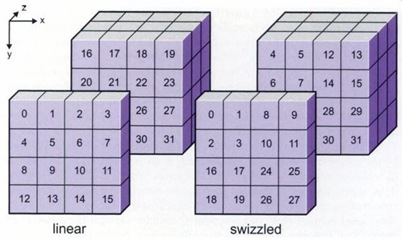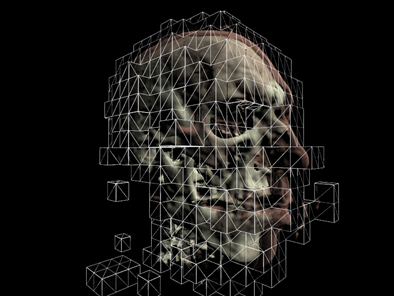## Wednesday, February 4, 2009

### Volume Rendering 201: Optimizations

The discussion on hand this time is optimizing the performance of the volume renderer. I’ll cover a few of these optimizations and provide a rough implementation of one of them.
Cache Efficiency and Memory AccessPicture from Real-time Volume Graphics.
Currently we load our volume data into a linear layout in memory. However, the ray that is cast through the volume is not likely to access neighboring voxels as we traverse it through the volume when the data is in a linear layout. But we can improve the cache efficiency by converting the layout to a block-based manor through swizzling. With the data in a block format, the GPU is more likely to cache neighboring voxels as we walk through the volume, which will lead to an increase in memory performance.
Empty-Space LeapingIn the previous samples we ray-casted against the entire bounding volume of the data set, even if we were just sampling samples with zero alpha along the way. But we can skip these samples all together, and only render parts of the volume that have a non-zero alpha in the transfer function. More on this in a bit.
Occlusion Culling
If we render the volume in a block-based fashion as above, and sort the blocks from front to back, we can use occlusion queries to determine which blocks are completely occluded by blocks in front of them. There are quite a few tutorials on occlusion queries on the net, including this one at ziggyware.
We can also boost performance by deferring the shading calculations. Instead shading every voxel during the ray-casting, we can just output the depth and color information into off-screen buffers. Then we render a full-screen quad and use the depth information to calculate normals in screen space and then continue to calculate the shading. Calculating normals this way also has the advantage of being smoother and have less artifacts that computing the gradients of the volume and storing them in a 3D texture. We also save memory this way since we don’t have to save the normals, only the isovalues in the 3D texture.
Image Downscaling
If the data we are rendering is low frequency (e.g. volumetric fog), we can render the volume into an off-screen buffer that is half the size of the window. Then we can up-scale this image during a final pass. This method is also included in the sample.
Implementing Empty-Space Leaping
To implement empty-space leaping we need to subdivide the volume into smaller volumes and we also need to determine if these smaller volumes have an opacity greater than zero. To subdivide the volume we follow an approach very similar to quadtree or octree construction. We start out with an original volume from [0, 0, 0] to [1, 1, 1]. The volume is then recursively subdivided until the volume width is say .1 (so we basically divide the volume along each dimension by 10). Here’s how we do that:
```private void RecursiveVolumeBuild(Cube C)
{
//stop when the current cube is 1/10 of the original volume
if (C.Width <= 0.1f)
{
//add the min/max vertex to the list

Vector3 min = new Vector3(C.X, C.Y, C.Z);

Vector3 max = new Vector3(C.X + C.Width, C.Y + C.Height, C.Z + C.Depth);

Vector3 scale = new Vector3(mWidth, mHeight, mDepth);

//additively sample the transfer function and check if there are any

//samples that are greater than zero

float opacity = SampleVolume3DWithTransfer(min * scale, max * scale);

if(opacity > 0.0f)

{

BoundingBox box = new BoundingBox(min, max);

//add the corners of the bounding box

Vector3[] corners = box.GetCorners();

for (int i = 0; i < 8; i++)

{

VertexPositionColor v;

v.Position = corners[i];

v.Color = Color.Blue;

}

}

return;
}

float newWidth = C.Width / 2f;
float newHeight = C.Height / 2f;
float newDepth = C.Depth / 2f;

///  SubGrid        r  c  d
///  Front:
///  Top-Left    :  0   0   0
///  Top-Right   :  0   1   0
///  Bottom-Left :  1   0   0
///  Bottom-Right:  1   1   0
///  Back:
///  Top-Left    :  0   0   1
///  Top-Right   :  0   1   1
///  Bottom-Left :  1   0   1
///  Bottom-Right:  1   1   1
for (float r = 0; r < 2; r++)
{
for (float c = 0; c < 2; c++)

{
for (float d = 0; d < 2; d++)

{

Cube cube = new Cube(C.Left + c * (newWidth),

C.Top + r * (newHeight),

C.Front + d * (newDepth),

newWidth,

newHeight,

newDepth);

RecursiveVolumeBuild(cube);

}

}
}
}```
To determine whether a sub-volume contains any samples that have opacity, we simply loop over the volume and additively sample the transfer function:
```private float SampleVolume3DWithTransfer(Vector3 min, Vector3 max)
{
float result = 0.0f;

for (int x = (int)min.X; x <= (int)max.X; x++)
{
for (int y = (int)min.Y; y <= (int)max.Y; y++)

{

for (int z = (int)min.Z; z <= (int)max.Z; z++)

{

//sample the volume to get the iso value

//it was stored [0, 1] so we need to scale to [0, 255]

int isovalue = (int)(sampleVolume(x, y, z) * 255.0f);

//accumulate the opacity from the transfer function

result += mTransferFunc[isovalue].W * 255.0f;

}

}

}

return result;
}```
Depending on the transfer function (a lot of zero opacity samples), this method can increase our performance by 50%.
Problems
Now, a problem that this method introduces is overdraw. You can see the effects of this when rotating the camera to view the back of the bear; here the frame rate drops considerably. To remedy this the sub-volumes need to be sorted front to back by their distance to the camera each time the view changes. I’ve left this as an exercise for the reader. The new demo implements empty space leaping and downscaling. And when rendering the teddy bear volume frame rates on my 8800GT are at about 190 FPS. Compare this to the the last demo from 102 at 30 FPS. All at a resolution of 800x600. Pretty good results! Next time I’ll be introducing soft shadows and translucent materials.

References: Real-time Volume Graphics